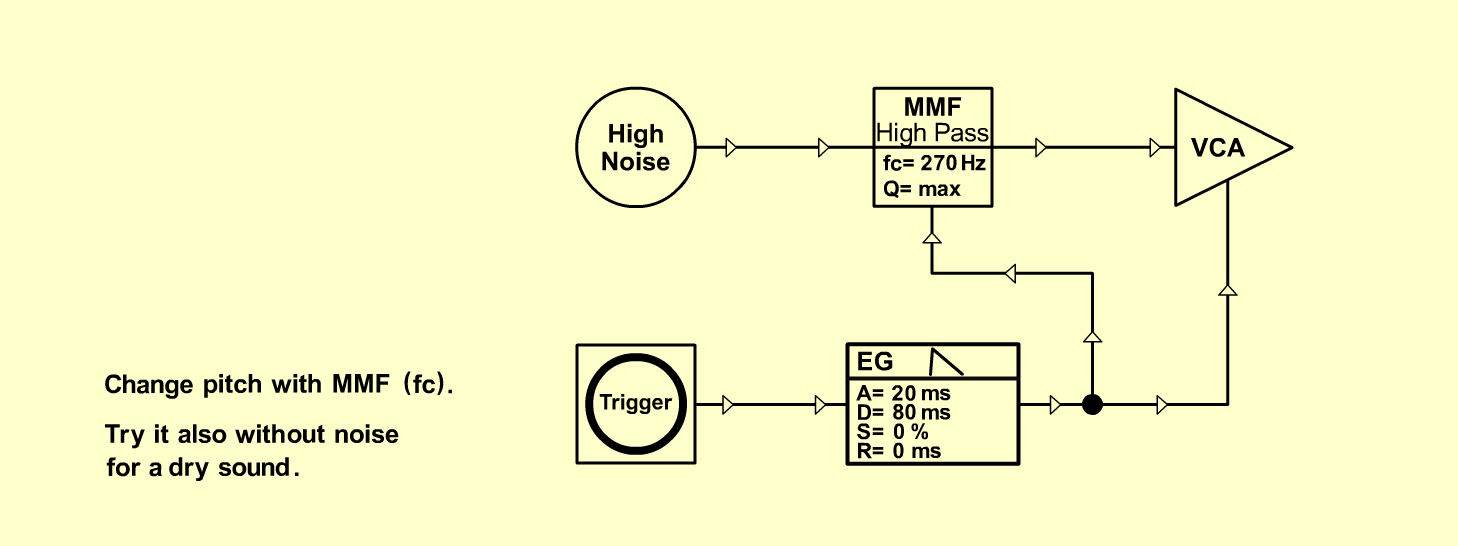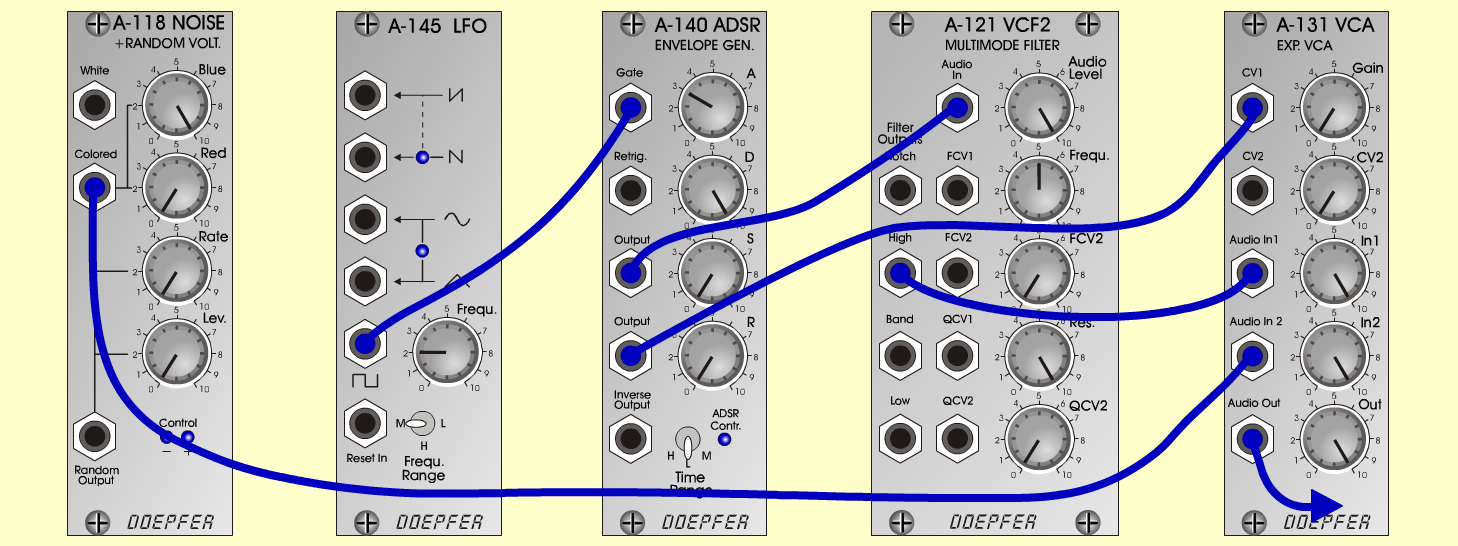SnareDrumD r u m sD o e p f e r A - 100 Connections: Settings: A-118 (Colored) <=> A-131 (Audio In 1)   A-121 (High) <=>  A-131 (Audio In 2)   A-140 (Output 1) <=>  A-121 (Audio In)   A-140 (Output 2) <=>  A-131 (CV 1)   Trigger: KB, Seq or   A-145 (Square) <=>  A-140 (Gate) A-118 (Blue = 10, Red = 0)   A-121 (Audio Level = 10, Freq = 5, Res = 10)   A-140 (A = 3, D = 10, S = 0, R = 0, Range = L)   A-131 (Gain = 0, Audio In 1 = 10, Audio In 2 = 10,   Audio Out = 10)   A-145 (Frq = 2, Range = M) Notes: Change pitch with A-121 (Freq).   Try it also without A-118 (Colored) for a dry   sound. Josef MuellerSound sample Snare Drum